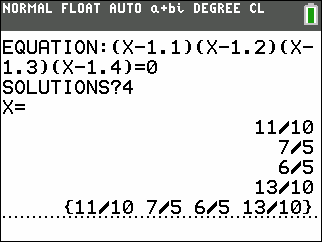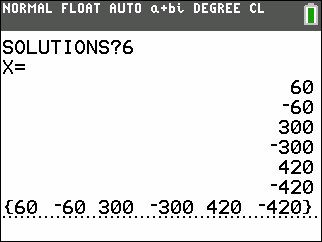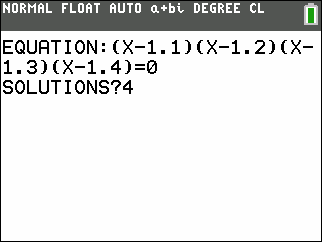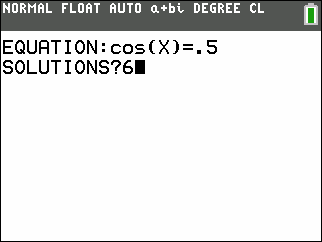Basics Archives Community Services ProgrammingHome :: Archives :: File Archives :: ALLSOLVEALLSOLVE

FILE INFORMATION

allsolve1.zip
 Filename allsolve1.zip (Download) Title ALLSOLVE Description ALLSOLVE: This is a multi purpose equation solver for real roots. It can solve a wide variety of equations. For example: log(X^2)=.4, abs((X-3)(X+2))=4, cos(X^2)=.5, (X-300)(X-400)(X-500)(X-600)=0, cos(X)=X^2+3X, (X+1.1)(X+1.2)(X+1.3)=0, abs(X^3-1)=7, (X^3-7)^(1/3)=X-1. First you enter the equation, then you enter the number of solutions that you search for. The program can find six solutions at the most. ALLSOLV1: This is the same program as the one above, except that you don't have to figure out how many roots the equation has. It shows them as it finds them. On the other hand it stops with an "ERROR: NO SIGN CHANGE", if there are less than six roots. Just press [ 1 ] then to quit. The roots are in list L1, and in the first six value memories. Authors Anders Tiberg (anders.tiberg@telia.com)Joe Erdeky (erdeky@inbox.com) Category TI-84 Plus C Silver Edition/CE BASIC Math Programs File Size 2,022 bytes File Date and Time Thu Sep 5 18:28:35 2013 Documentation Included? Yes

SCREEN SHOTSREVIEWS

There are no reviews for this file.

LEAVE FEEDBACK

Questions, comments, and problems regarding the file itself should be sent directly to the author(s) listed above.

Report inappropriate or miscategorized file (requires an account; or you may email us directly)